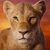# Believe It or Not, Only 10% Of Adults Can Pass This Fractions Test

## Fractions may appear to be easy at first glance, but they can actually be pretty complicated! Can you pass this fractions test?Created by Lea Abelson
On Feb 25, 2023

Is math your thing? Do you consider yourself highly intelligent in math subjects like geometry, algebra, and even fractions? It's crazy but most adults can't even get an average score on this fractions test. See if you can guess the decimal number or fraction from the value given.

1 / 10

6/7

What is the decimal number?

2 / 10

0.999

What is the fraction?

3 / 10

4/5

What is the decimal number?

4 / 10

1.44

What is the fraction?

5 / 10

33/44

What is the decimal number?

6 / 10

9.98
﻿
What is the fraction?

7 / 10

4/3

What is the decimal number?

8 / 10

8.66

What is the fraction?

9 / 10

33/9

What is the decimal?

10 / 10

7/5

What is the decimal number?

10
Questions left

### Which math subject could you totally master?

Calculating results
These are 10 of the World CRAZIEST Ice Cream Flavors
Created by Tal Garner
On Nov 18, 2021